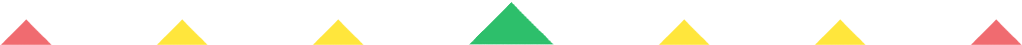Player 1
vs
Player 2
0:00
Opponent history
x
xCorrect
0
Wrong
0
Correct!
Wrong!
Help Sheet for

###• Double 1 = 2
• Double 2 = 4
• Double 3 = 6
• Double 4 = 8
• Double 5 = 10
• Double 6 = 12
• Double 7 = 14
• Double 8 = 16
• Double 9 = 18
• Double 10 = 20
• Double 11 = 22
• Double 12 = 24